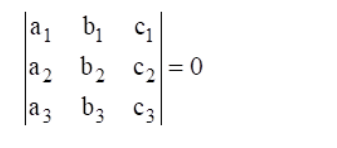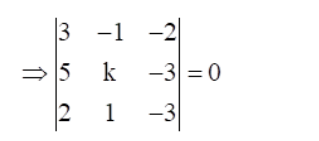# Find the value of k so that the lines`
Question:

Find the value of k so that the lines $3 x-y-2=0,5 x+k y-3=0$ and $2 x+y-3 = 0 are concurrent. Solution: Given that$3 x-y-2=0$,$5 x+k y-3=0$and$2 x+y-3=0$are concurrent We know that, The lines$a_{1} x+b_{1} y+c_{1}=0, a_{1} x+b_{1} y+c_{1}=0$and$a_{1} x+b_{1} y+c_{1}=0$are concurrent ifIt is given that the given lines are concurrent.Now, expanding along first row, we get$\Rightarrow 3[(k)(-3)-(-3)(1)]-(-1)[(5)(-3)-(-3)(2)]+(-2)[5-2 k]=0\Rightarrow 3[-3 k+3]+1[-15+6]-2[5-2 k]=0\Rightarrow-9 k+9-9-10+4 k=0\Rightarrow-5 k-10=0\Rightarrow-5 k=10\Rightarrow k=-2\$

Hence, the value of k = -2